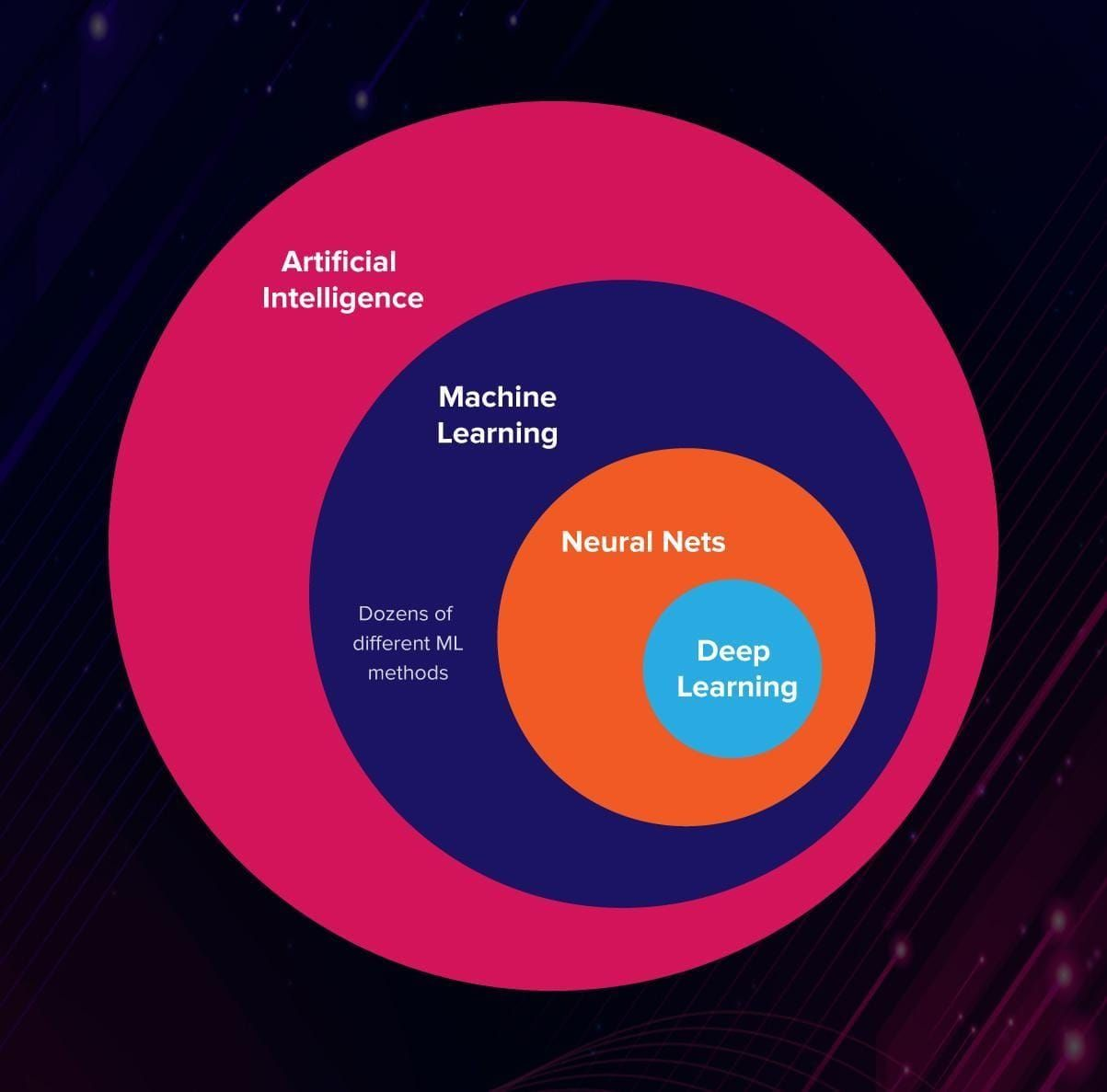Khalid M. Elboray

NNFS with Raku _ Part 1

In this series of posts i will try to implement some or hopefully all of the code introduced in the book Neural Networks from Scratch in Python.

Neural Networks

Neural Networks or Artificial Neural Networks reflect the behavior of the behavior of the human brain, allowing computer programs to solve common problems in the fields of AI, machine learning and deep learning.

What are neural networksArtificial neural networks are inspired by the architecture of the human brain to perform tasks that conventional algorithms has little success with.

ANNs are comprised of a node layers, containing an input layer, one or more hidden layers, and an output layer. Each node, or artificial neuron, connects to another and has an associated weight and threshold. If the output of any individual node is above the specified threshold value, the node is activated, sending data to the next layer of the network, Otherwise, no data is passed along the next layer of the network.

• A neuron acts as an all-or-one switch, that outputs an action potential or no output.

• The connection between each neuron is attributed a weight acting on the data flowing.The Mathematics of a neuron (The Perceptron)

The perceptron is an algorithm for learning a binary classifier called a threshold function: a function that maps its input $x$ (a real-valued vector) to an output value $f(x)$ (a single binary value):

$f(x) = \begin{cases} 1 & if \ \ w \cdot x + b > 0 \\ \\ 0 & otherwise \end{cases}$

where $w$ is a vector of real-valued weights and $w \cdot x$ is the dot product

$w \cdot x = \sum_{i=1}^m \ w_ix_i$

The value of $f(x)$ (0 or 1) is used to classify $x$ as either a positive or a negative instance.

Coding Our First Neuron

At first we will be doing things from scratch with the fact that Raku is quite capable of doing our math stuff.

Most of our work will be about Matrices and Vectors and operations on them which again is easily done with Raku. But performance can be important for some tasks so we will later show a faster and more efficient alternative Libgsl.

A single Neuron

As we discussed for each neuron there is some inputs. In most cases we initialize the parameters in neural networks, in a neural networks we will have weights initialized randomly, and biases set as zero to start with. The input will be either the training data or the outputs of neurons from a previous layer in the network. We will start with making up some values as input for our neuron.

Each input needs a weight associated with it. Inputs are the data passed into the model to get the desired outputs, while the weights are the parameters that will be tuned during the model training phase, biases will also change during training. The values of weights and biases are what get trained and they are what makes our model work (or not work). Again we will make up some weights for now. Let’s say that the first input, at index 0 , which is 1.2 has the weight of 3.1 , the second input has a weight of 2.1 and so on. Our input and weights lists should be:

Next, we need a bias, since we are working with a single neuron we only need one bias and we will randomly set it to the value of 2.

The neuron will sum each input multiplied by input’s weight, then adds the bias, which can be calculated like:

Our code up to this point:

Which can be shortened (using the Reduction metaoperator [+] and the Hyper_operator >>*<< to:

That’s it for now, in the coming part we will implement a layer of neurons and re-implement our code using Libgsl.